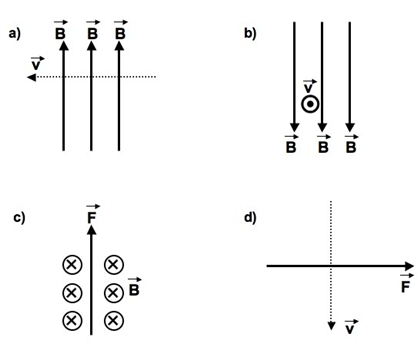# Problem: 1. In the arrangements shown, assume a positive electrical charge is moving with velocity v through a magnetic field B. Answer the following questions about theindicated Figure:Figure (a): Show the direction of the force.Figure (b): Show the direction of the force.Figure (c): Show the direction of the velocity.Figure (d): Show the direction of the magnetic field.2. Repeat each part of Question #1 for a negative charge.

###### FREE Expert Solution

The magnitude of magnetic force:

$\overline{){{\mathbf{F}}}_{{\mathbf{B}}}{\mathbf{=}}{\mathbf{q}}\stackrel{\mathbf{⇀}}{\mathbf{v}}\stackrel{\mathbf{⇀}}{\mathbf{B}}{\mathbf{s}}{\mathbf{i}}{\mathbf{n}}{\mathbf{\theta }}}$

96% (353 ratings)###### Problem Details1. In the arrangements shown, assume a positive electrical charge is moving with velocity v through a magnetic field B. Answer the following questions about the
indicated Figure:

Figure (a): Show the direction of the force.

Figure (b): Show the direction of the force.

Figure (c): Show the direction of the velocity.

Figure (d): Show the direction of the magnetic field.

2. Repeat each part of Question #1 for a negative charge.